0

# 3D Seawater Wave Flooring Sticker Wallpaper

Custom Floor Mural Wallpaper (Self-adhesive PVC Sticker)

#wallpaper #nordicinterior #interiordesign #diyhomedecor #realestate #architecture

How to place the order

Step 1:  Measure your floor dimension: Length * Width  = Square meter

For example: You need the size is 3 meters in length, 2 meters in width.

According to area size is: 3 M * 2 M = 6 Square meters.

Step 2:   Choose the right quantity in the order.

If you need 6 square meters, Please place the order of 6 square meter.

If you need 7.1- 7.9 square meter, Please place the order of 8 square meter.

Step 3:  Remark the exact floor mural wallpaper length and width.

Please provide me the exact mural wallpaper length and width below the order within 24 hours.

If we haven't got your exact size after 24 hours,We will arrange them for producing according to the default size.

If you need 6 square meters, please choose "1 square meter" and "Quantity 6" here.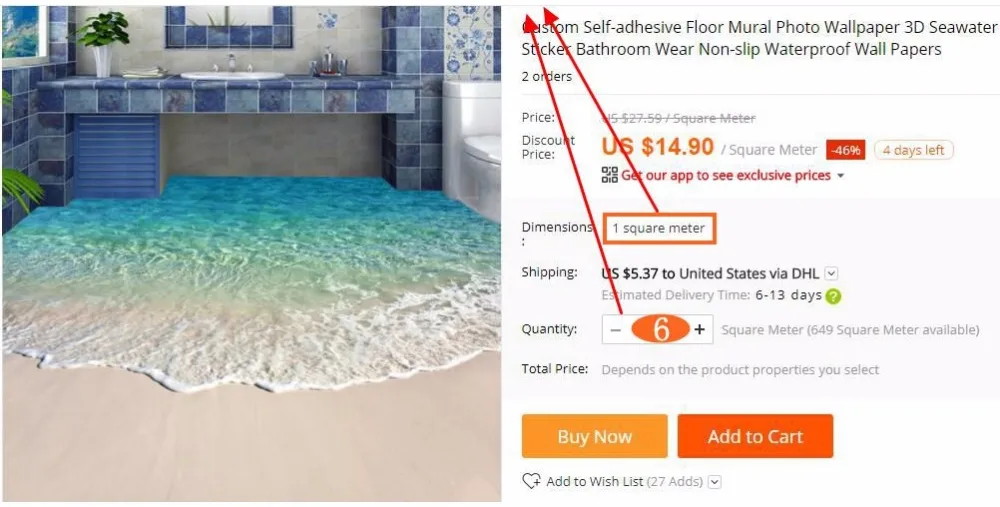Default size :

Quantity 1 :     1 square meter=140cm(L) x 70cm(W) (4'7" x 2'4") ft

Quantity 2:      2 square meter=200cm(L) x 100cm(W) (6'7" x 3'3") ft

Quantity 3:      3 square meter=208cm(L) x 146cm(W) (6'9" x 4'9") ft

Quantity 4:      4 square meter=200cm(L) x 200cm(W) (6'7" x 6'7") ft

Quantity 5:      5 square meter=250cm(L) x 200cm(W) (8'2" x 6'7") ft

Quantity 6:      6 square meter=300cm(L) x 200cm(W) (9'10" x 6'7") ft

Quantity 7:      7 square meter=300cm(L) x 250cm(W) (9'10" x 8'2") ft

Quantity 8:      8 square meter=400cm(L) x 200cm(W) (13'1" x 6'7") ft

Quantity 9:      9 square meter=350cm(L) x 260cm(W) (11'5" x 8'6") ft

Quantity 10:   10 square meter=360cm(L) x 280cm(W) (11'10" x 9'2") ft

Quantity 12:   12 square meter=400cm(L) x 300cm(W) (13'1" x 9'10") ft

Quantity 16:   16 square meter=500cm(L) x 320cm(W) (16'5" x 10'6") ft

Product show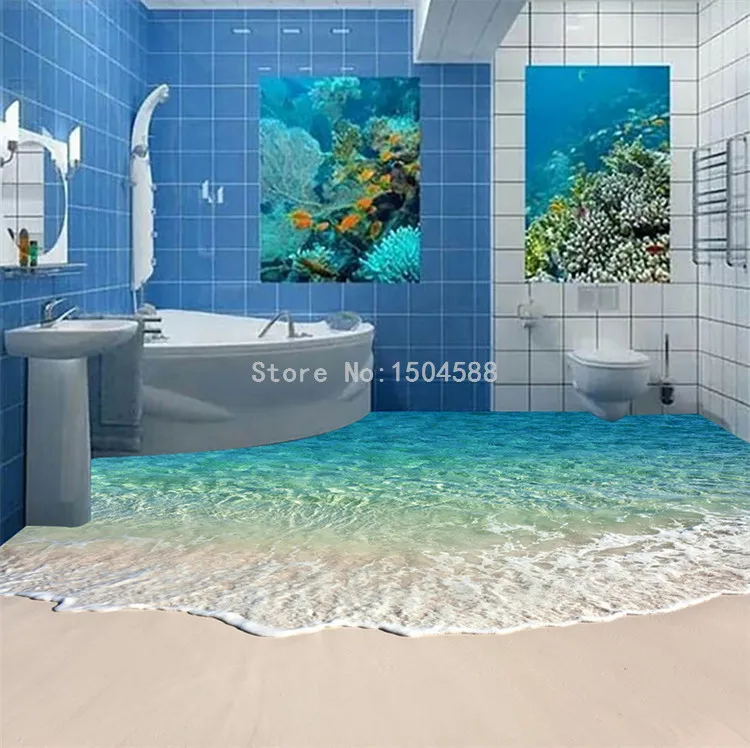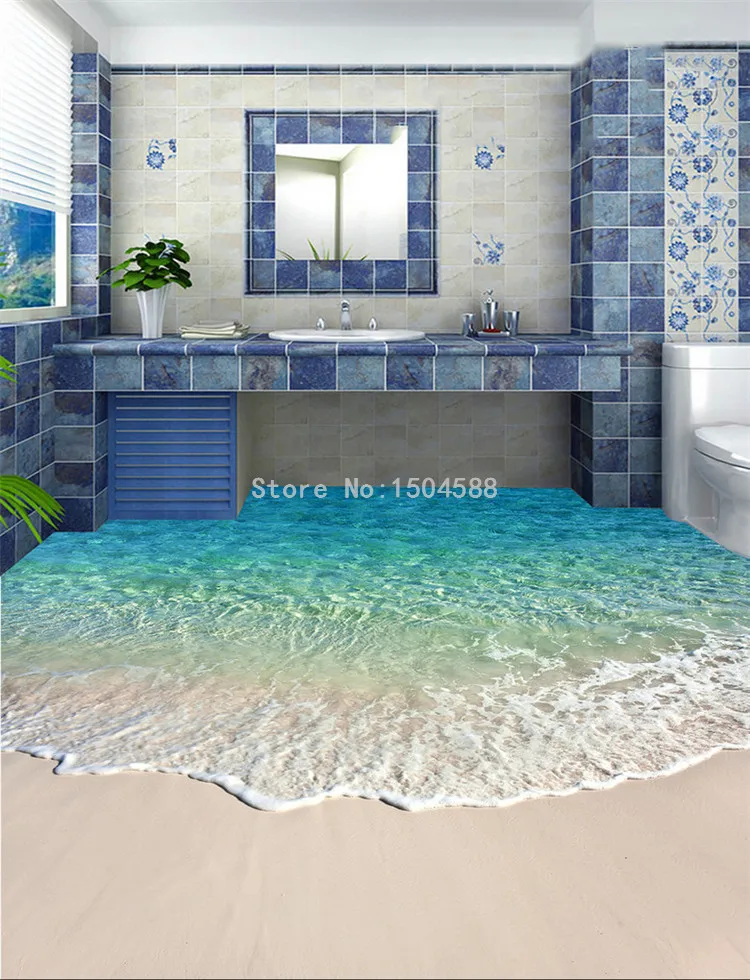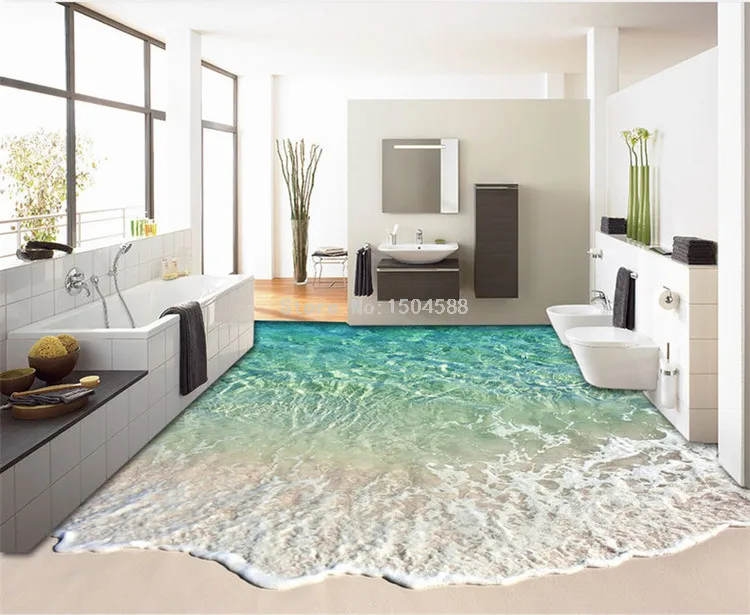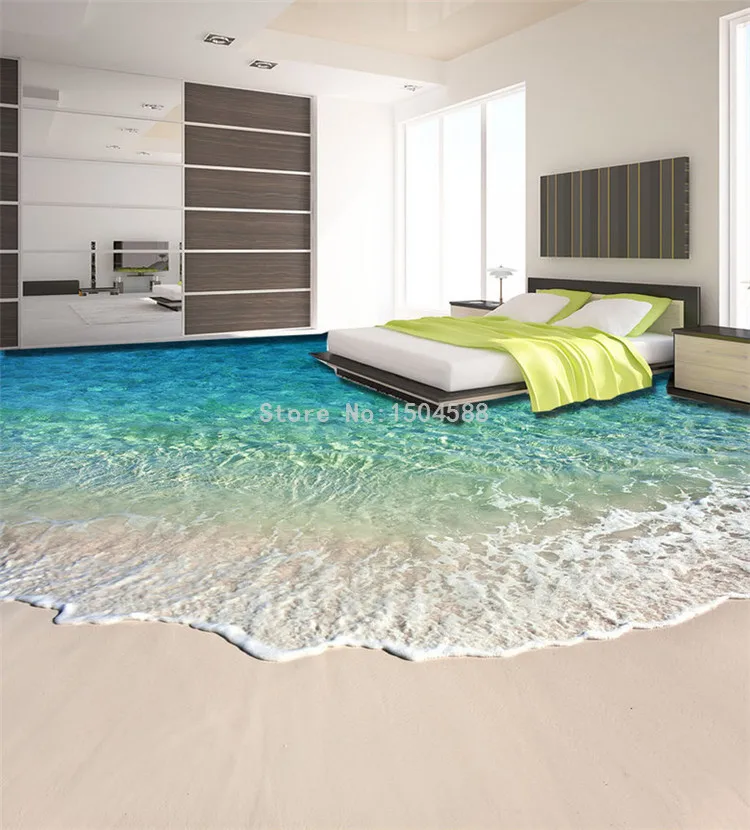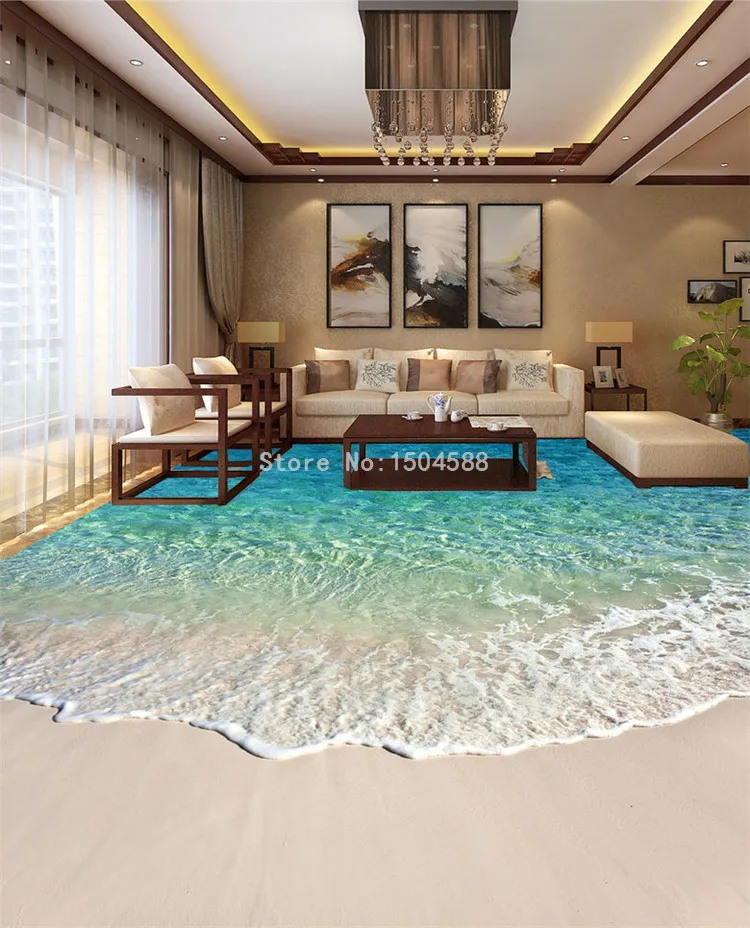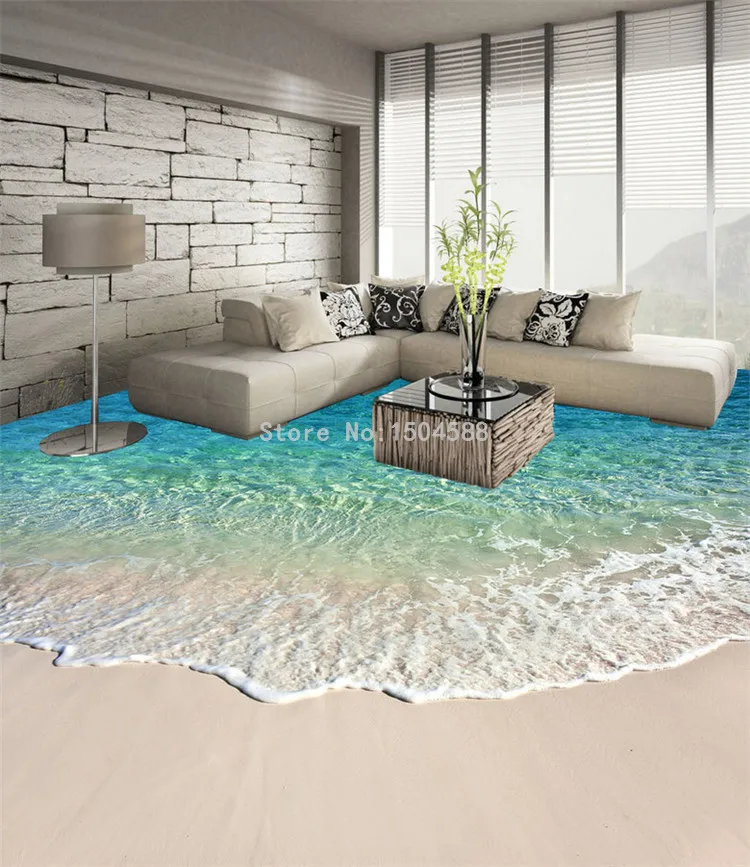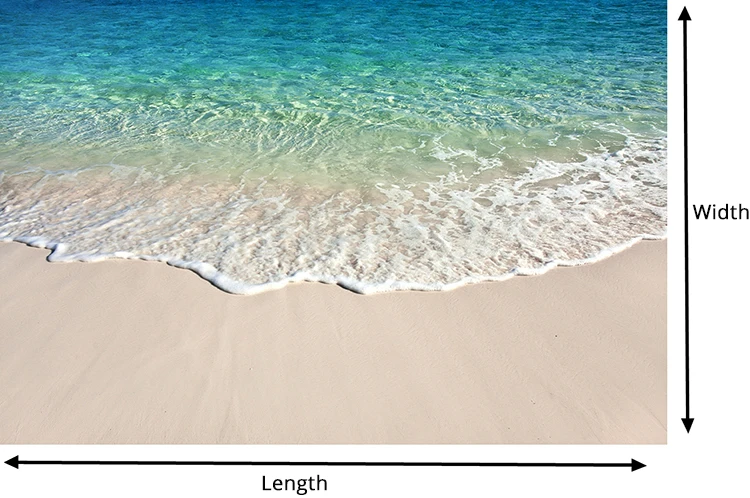Super thick. Waterproof.  Skidproof  PVC Self-adhesive Floor Sticker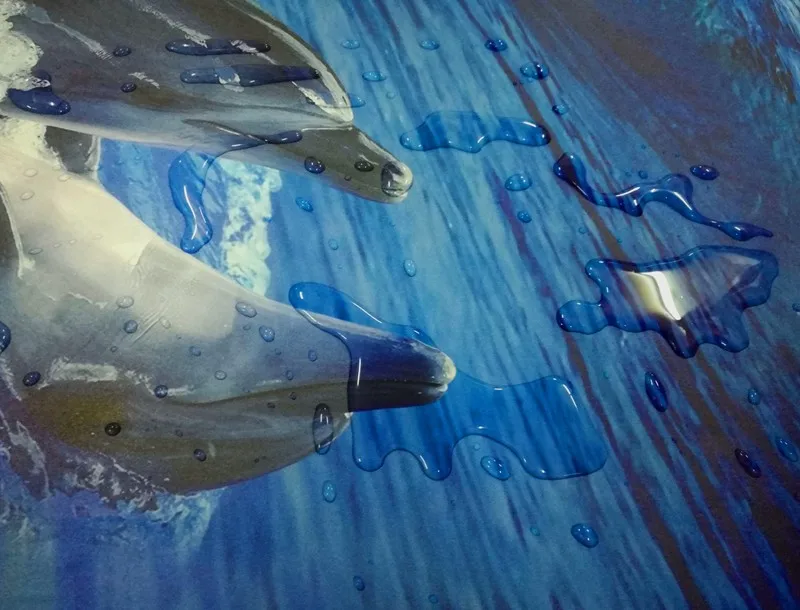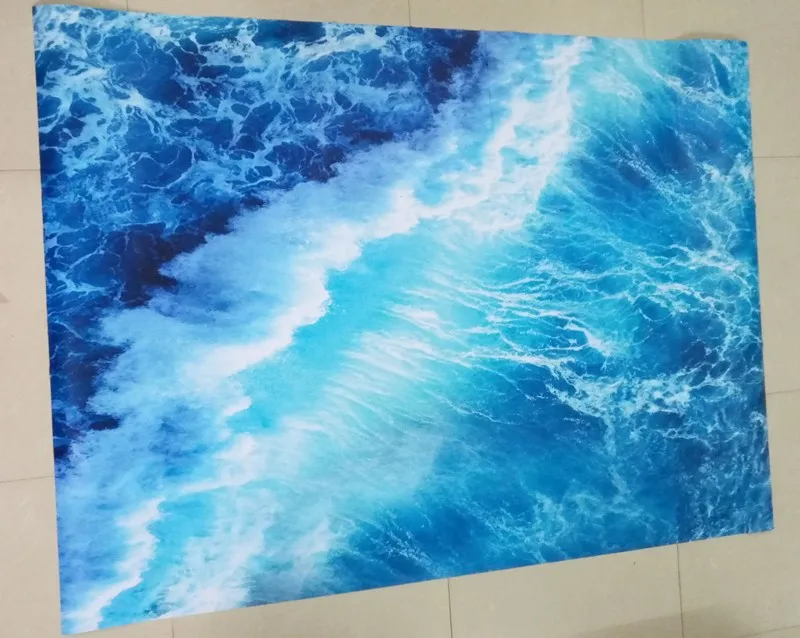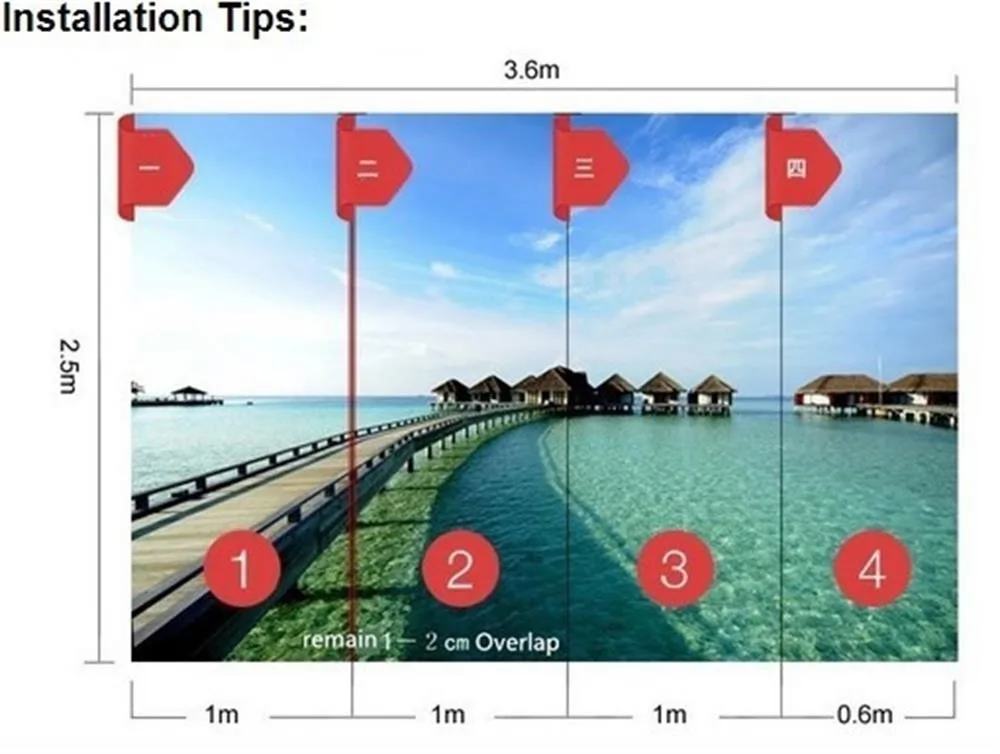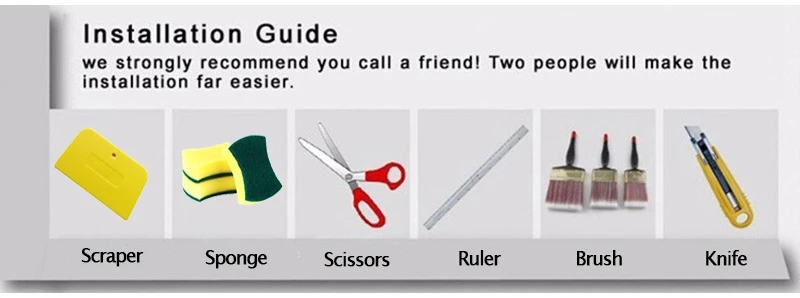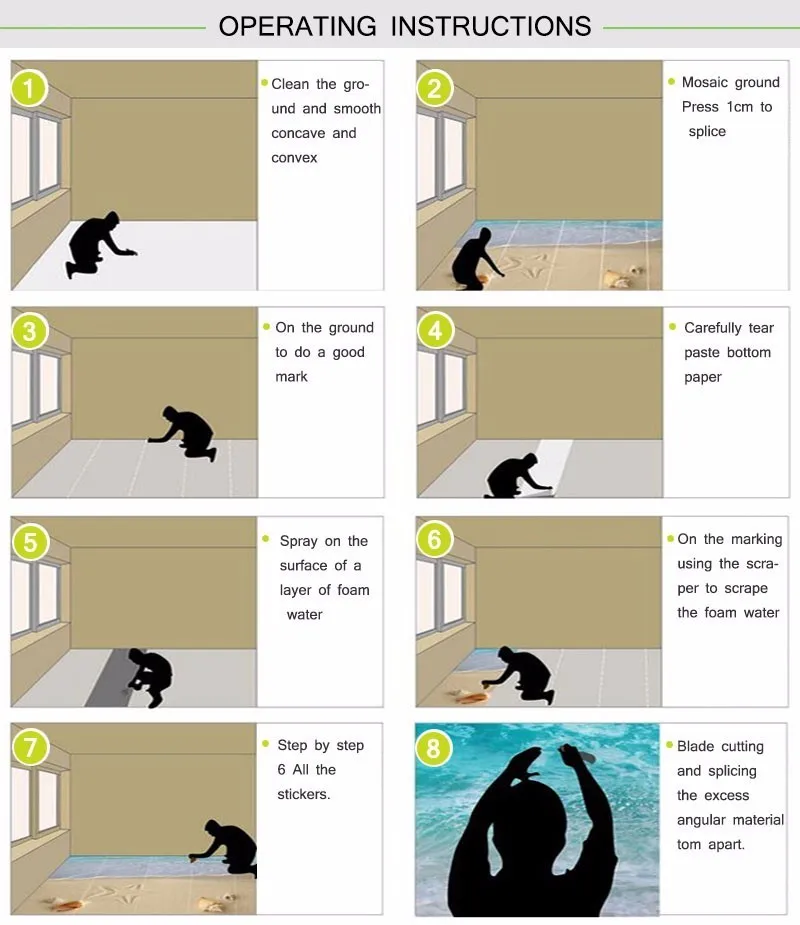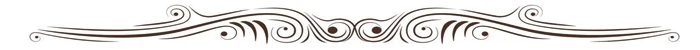☆ Thank You!☆